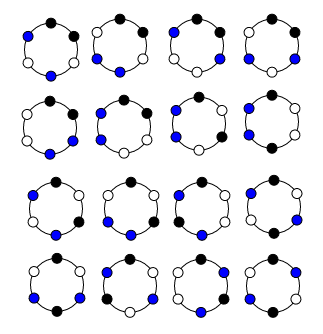Probability of $6$ balls around a circle

Problem: $2$ blue, $2$ red, $2$ white balls are arranged around a circle randomly. What is the probability that the same color balls will always neighboring?

1. I did'n find correct last word for the problem: '...always neighboring? ...always together? ...always side by side?' I hope that the problem statement clearly.

2. I have some solutions of the problem. I think that first solution is correct. But I'm not sure, Which is correct?

Solution 1: There is no identical object in the probability. By circular permutation, number of elements of sample space is $(6-1)!=5!=120$. Number of elements of the given event is $(3-1)!\cdot 2\cdot 2 \cdot 2 = 16$ Hence, probability of given event $$p= \dfrac{16}{120} = \dfrac{2}{15}$$

Alternatively Solution 2: There is no identical object and there is no identical location in the probability. That is, we can't use circular permutation and identical objects. So, number of elements of sample space is $6!=720$. Number of the given event is $3!\cdot 2\cdot 2 \cdot 2 = 48$. Probability of given event $$p= \dfrac{48}{720} = \dfrac{1}{15}$$

Alternatively Solution 3: We have to take identical object same color balls. Easily, we can calculate number of elements of sample space that $16$. Also, Number of elements of the given event is $2$. Therefore, probability of given event $$p= \dfrac{2}{16} = \dfrac{1}{8}$$

$16$ elements of the sample space for identical objects:Thanks for your interest.

Added later: Your edit to the question with a diagram has raised an issue about the first part of my earlier response

I initially agreed with your first result, saying:

• There are $2$ patterns with the colours together (clockwise either blue-red-white or blue-white-red)
• There are $\dfrac{6!}{6\times2^3}=15$ equally probable ways of arranging the balls (dividing by $6$ because of rotational symmetry, and $2^3$ because of the three pairs of colours)

As your diagram shows, there are in fact $16$ possible patterns after taking account of rotations and double colours rather than $15$. To complicate matters, these $16$ are not equally likely and that is the problem with your third alternative.

Most of the patterns can occur $6 \times 2^3=48$ different ways. But two of them cannot, namely those where opposite balls are the same colour (the final patterns in your third and fourth rows); because they are rotationally symmetric to themselves through half a circle, they can only occur $24$ different ways and so should only have half a weight in the calculation. That would make the probability $\dfrac{2 \times 1}{14\times 1 + 2 \times \frac12}= \dfrac2{15}$

Your second alternative is missing half the possibilities. If all the balls can be individually identified and all the positions can be identified then I would have thought the numerator would counting choices clockwise from a given position be $6 \times 1 \times 4 \times 1 \times 2 \times 1 + 6 \times 4 \times 1 \times 2 \times 1 \times 1 = 96$.

The denominator is then $6!=720$ and so the result is $\dfrac{96}{720}=\dfrac{2}{15}$ again

• Thanks for your interest, Henry. I would agree with your second method. But; in your first solution method, sample space has $16$ elements. I draw all cases in my third solution. Unfortunately, your first solution method may has a mistake. – scarface Jan 29 '18 at 20:13
• That is an excellent point. I have edited the answer to cover it - it turns out that $2$ of your $16$ possibilities are half as likely to appear as the others – Henry Jan 29 '18 at 22:40
• Thank you very much. I understand from your solutions that there is no identical objects in the probability theory. May you give me some links or source books photos about this fact? Best regards – scarface Feb 1 '18 at 12:10
• Hi @Henry , Thank you very much. I understand from your solutions that there is no identical objects in the probability theory. May you give me some links or source books photos about this fact? Best regards – scarface Feb 17 '18 at 22:11
• Until you reach some of the oddities of quantum theory, it is difficult to have identical items: two balls are at least in different places at the same time – Henry Feb 19 '18 at 0:38

We will first calculate the number of ways the adjacent balls are not of the same colour. We need to visualise this into two sets of 3 balls, each set having one of the 3 colours. For each of the 3 possible ways to arrange each set of 3 balls(since the table is round), there are 2 ways to arrange the other 3 balls. We have $2\times3=6$ ways here.

Total number of ways is $\frac{6!}{2!\times2!\times2!}=90$.

The ways to put same-coloured balls together is $\frac{90-6}{90}=\frac{14}{15}$. In your answer, you might have forgotten that you were counting for adjacent balls not of the same colour.